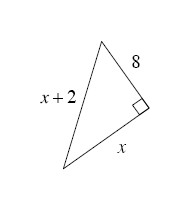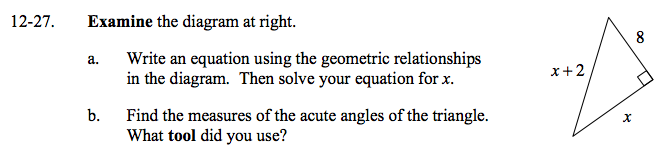### Home > GC > Chapter 12 > Lesson 12.1.3 > Problem12-27

12-27.
1. the diagram below. Homework Help ✎1. Write an equation using the geometric relationships in the diagram. Then solve your equation for x.

2. Find the measures of the acute angles of the triangle. What tool did you use?Use the Pythagorean Theorem.

x² + 8² = (x + 2)²
x² + 64 = x² + 4x + 4
60 = 4x
x = 15

What trig ratio can you use to find one of the angles? Once you have two angles, what relationship can you use to find the last angle?

28.1°, 61.9°